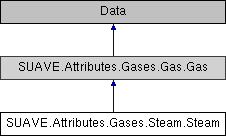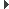SUAVE  2.5.2 An Aerospace Vehicle Environment for Designing Future AircraftSUAVE.Attributes.Gases.Steam.Steam Class Reference
Inheritance diagram for SUAVE.Attributes.Gases.Steam.Steam:## Public Member Functions

def __defaults__ (self)

def compute_density (self, T=300, p=101325)

def compute_speed_of_sound (self, T=300, p=101325, variable_gamma=False)

def compute_cv (self, T=300, p=101325)

def compute_cp (self, T=300, p=101325)

def compute_gamma (self, T=300, p=101325)

def compute_absolute_viscosity (self, T=300, p=101325)

## Public Attributes

tag

molecular_mass

gas_specific_constantPublic Attributes inherited from SUAVE.Attributes.Gases.Gas.Gas
tag

molecular_mass

gas_specific_constant

composition

## Detailed Description

```Holds constants and functions that compute gas properties for steam.

Assumptions:
None

Source:
None
```

## ◆ __defaults__()

 def SUAVE.Attributes.Gases.Steam.Steam.__defaults__ ( self )
```This sets the default values.

Assumptions:
None

Source:
Values commonly available

Inputs:
None

Outputs:
None

Properties Used:
None
```

Reimplemented from SUAVE.Attributes.Gases.Gas.Gas.

## ◆ compute_absolute_viscosity()

 def SUAVE.Attributes.Gases.Steam.Steam.compute_absolute_viscosity ( self, T = `300`, p = `101325` )
```Gives constant absolute viscosity of 1e-6
WARNING: this value appears to be incorrect

Assumptions:
Constant value

Source:
Common value

Inputs:
None

Outputs:
mu0        [kg/(m-s)]

Properties Used:
None
```

## ◆ compute_cp()

 def SUAVE.Attributes.Gases.Steam.Steam.compute_cp ( self, T = `300`, p = `101325` )
```Computes Cp by 3rd-order polynomial data fit:
cp(T) = c1*T^3 + c2*T^2 + c3*T + c4

Assumptions:
300 K < T < 1500 K

Source:
Unknown, possibly Combustion Technologies for a Clean Environment
(Energy, Combustion and the Environment), Jun 15, 1995, Carvalhoc

Inputs:
T              [K]       - Temperature
p              [Pa]      - Pressure

Outputs:
cp             [J/kg-K]

Properties Used:
None
```

## ◆ compute_cv()

 def SUAVE.Attributes.Gases.Steam.Steam.compute_cv ( self, T = `300`, p = `101325` )
```Stub for computing Cv - not functional
```

## ◆ compute_density()

 def SUAVE.Attributes.Gases.Steam.Steam.compute_density ( self, T = `300`, p = `101325` )
```Computes air density given temperature and pressure

Assumptions:
Ideal gas

Source:
Common equation

Inputs:
T         [K]  - Temperature
p         [Pa] - Pressure

Outputs:
density   [kg/m^3]

Properties Used:
self.gas_specific_constant
```

## ◆ compute_gamma()

 def SUAVE.Attributes.Gases.Steam.Steam.compute_gamma ( self, T = `300`, p = `101325` )
```Gives constant gamma of 1.33

Assumptions:
233 K < T < 1273 K

Source:
Common value

Inputs:
None

Outputs:
g              [-]

Properties Used:
None
```

## ◆ compute_speed_of_sound()

 def SUAVE.Attributes.Gases.Steam.Steam.compute_speed_of_sound ( self, T = `300`, p = `101325`, variable_gamma = `False` )
```Computes speed of sound given temperature and pressure

Assumptions:
Ideal gas with gamma = 1.33 if variable gamma is False

Source:
Common equation

Inputs:
T              [K]       - Temperature
p              [Pa]      - Pressure
variable_gamma <boolean> - Determines if gamma is computed

Outputs:
speed of sound [m/s]

Properties Used:
self.compute_gamma() (if variable gamma is True)
self.gas_specific_constant
```

The documentation for this class was generated from the following file:
• /Users/emiliobotero/Dropbox/SUAVE/SUAVE/trunk/SUAVE/Attributes/Gases/Steam.py